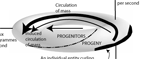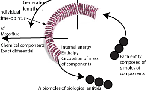﻿ Relativity and Biology | Engenetics: The science of species (by Kofi Busia)

# Relativity in biology and ecology

Einstein's special theory of relativity, which incorporates the equivalence of all mass and energy, shows that ‘species’ is a manifestion of that theory. Species may be a manifestion of the special theory, but the process of ‘speciation’ is then a manifestation of the general theory of relativity. (You can find the full analysis and explanation here). We demonstrate this by first taking up the equilibrium age distribution population for a given species. We then use the Einstein formula E=mc2 from the special theory to convert our equilibrium distribution population's entire rest mass into energy. We then add to that all the population's chemical bond, thermal, mechanical, and other energies. We place the entire sum in element T00 in biology's “Haeckel tensor”, which is the equivalent of the general theory's stress-energy tensor (see underneath). This sum of all energies placed in T00 becomes that population's “Haeckel potential”, ηH. Using standard mathemtical procedures we then allocate that entire relativistic energy firstly as an energy flux to the three elements to the right of T00, which are the three columns headed by T01, T02 and T03. And then secondly, we allocate them to the three elements underneath T00, as the intensity of the general theory's momentum density—and so as a momentum flux—to the three rows headed by T10, T20 and T30. Those three rows and columns contained by this distribution of the energy in T00 are now biology's “Owen tensor”, and the equivalent of general relativity's “Cauchy tensor”. The three rightmost elements in the top row of the Haeckel tensor now head up, or oversee, the three columns in the contained Owen tensor. They are measurable, spacelike, biological masses and energies. Those three columns can each be measured (1) absolutely; and (2) relatively. These two complementary sets of spacelike measures are linked by the Lorentz transformations as used in special relativity. The element T00 provides the mass-energy distributed across the Owen tensor's three columns. When those three columns T01, T02 and T03 are measured absolutely, they are the three constraints of (i) constant propagation (measured in joules per time increment over the generation (e.g. per second)), (ii) constant size (measured in joules per unit population size which is per biomole); and (iii) constant equivalence (measured as watts per kilogramme). When the same three columns are measured relatively, they invoke the Lorentz transformations and provide a set of unit normal vectors. They then become the three engenetic burdens of (i) fertility, φ; (ii) components mass, κ; and (iii) conformation, χ. It is also element T00 in the Haeckel tensor that provides the energy for the leftmost column. The three elements in their turn head up the three rows in the contained Owen tensor. When those three elements at the heads of the rows T10, T20 and T30 are in their turn measured absolutely, they are the three “materials fluxes” responsible for the spacelike materials distribution that we converted, above, into pure energy through E=mc2. These momentum density materials fluxes are (i) the number flux, Q, which is the discrete number of entities to be maintained in biomoles per second; (ii) the mass flux, M, which is the mass of chemical components the population maintains in kilogrammes per second; and (iii) the energy flux, P, of chemical bond energies used to bind and maintain those entities and components, measured in watts or joules per second. These various masses and energies must be distributed timelike across the entire generation length, T. This generation length can also be measured both absolutely and relatively. It can be measured for each population in seconds; but it can also be measured relatively for all populations from zero to unity. The former gives the infinitesimal increments dT, while the latter gives the increments dθ and dτ which are all measurable in all populations. The larger Haeckel tensor's four row values T00, T10, T20 and T30 are then the four evolutionary potentials. These are: (i) the Haeckel potential, ηH, which distributes the entire mass-energy materially and so spacelike, establishing the timelike rates in mass-energy at every point across the generation length; (ii) the Darwin potential, ηD, which distributes that same mass-energy materially and spacelike over the population numbers which are then maintained at and with a timelike rate; (iii) the Mendel potential, ηM, which distributes the same mass-energy spacelike and materially, and into the moles of the various chemical components the entities use, all again with a timelike rate; and (iv) the Gibbs potential, ηG, which is the Gibbs energy that distributes the relevant mass-energy spacelike across the given chemical bonds that form and shape the entities and compounds, as well as distributing it across all thermal and other energies, all with a timelike rate. As is standard in general relativity, these four rows and four columns intersect in the Haeckel tensor's four elements, and are the equivalent of the general theory's stress-energy tensor (see underneath) to produce all observed values in any population. The demonstrated and experimental measurements made for the test species Brassica rapa are available elsewhere on this site. The sum of the mass-energies intersecting spacelike and timelike in T11, T22 and T33 are general relativity's “normal pressures”. The sum of those normal pressures is the “Ricci scalar curvature”; or the “trace”, or the “contraction” of the “Ricci tensor”. The Ricci scalar and tensor govern all the materials mass and energy observed throughout the population. The Ricci scalar is the population's essential development, λ. Its value is unique to every population. The sum of the mass-energies in all the other intersecting elements in the Haeckel tensor, i.e. off the main diagonal, is the “shear pressure” and the “trace-free” “Weyl tensor”. It is the population's compensatory development, L. A biological population can only be free from Darwinian evolution if its compensatory development, L, is zero. But this requires that, in the special theory, the axes of the Minkowski light cone become parallel, and that spacetime become flat and empty … which is impossible. Einstein's general theory proves that all masses and all energies curve and bend space and time. The degree of curvature depends entirely upon the spacelike and timelike distribution of mass-energy. This is as numbers of entities in the population, along with their masses in kilogrammes and their energies in joules. Therefore, a flat spacetime is impossible and a population free from Darwinian competition and evolution is also simply impossible. Evolution is simply the natural movement of energy along the intrinsically curved axes of spacetime. The values by which any population curves and bends light in the spacetime it creates about itself is soon calculated. The values for Brassica rapa were determined by experiment and are available elsewhere on this site. The special theory then shows that if a given population can maintain constant values for its essential and compensatory developments, over time, then it can establish a species. Speciation occurs, under the general theory, when the variations in mass and energy to which all biological organisms are subject cause space and time to bend and curve axes in spacetime until—under the special theory—that population can maintain a different set of intrinsic curvatures of mass-energy and spacetime, and a new set of spacelike and timelike values and coordinates.

# The Haeckel (or stress-energy) tensor

 H Haeckel   T00   potential, ηH a e c k e l p o t e n t i a l ηH Three engenetic burdens (relative measures) Engenetic burden of fertility, φ Engenetic burden of components mass, κ Engenetic burden of conformation, χ Three constraints (absolute measures) Constraint of constant propagation (joules per generational increment) Constraint of constant size (joules per biomole) Constraint of constant equivalence (watts per kilogramme) n entities q molecules w bonding Four potentials (relative measures) T01 T02 T03 Darwin Three fluxes (absolute measures) Number flux, Q (biomoles per second) n T10 T11 T12 T13 potential, ηD entities Pronumeracy Anumeracy Anumeracy Mendel Mass flux, M (kilogrammes per second) q T20 T21 T22 T23 Compensatory potential, ηM molecules Abundance Probundance Abundance Development Gibbs Energy flux, P, (joules per second) w T30 T31 T32 T33 potential, ηG bonding Accreativity Accreativity Procreativity Compensatory Essential Development Development

### Ecology is “the study of the processes systems use to replace their internal energy”.

#### pdt + mdt = dh + du = δq; m > 0 (See explanation of terms and variables)

G  O    T  O    L  A  W  SLAW 1: The law of existence
n ≥ 1; δW = (δQ - dU) > 0; m → ∞; > 0

LAW 2: The law of equivalence
[(δW1 = δW2) ∧ (δW2 = δW3)] ⇒ (δW1 = δW3)

LAW 3: The law of diversity
A → 0; FM

LAW 4: The law of reproduction
[(dm̅/dt ≤ 0) ∧ ( > 0)] ⇒ [(dn/dt ≥ 0) ∧ (dA/dt > 0)]MAXIM 1: The maxim of dissipation
[Darwin's theory of competition]
M = nm̅;   ∫dm < 0;   ∇ • M → 0

MAXIM 2: The maxim of number
∇ • H = H/n =

MAXIM 3: The maxim of succession
[Darwin's theory of evolution]
∇ x M = ∂/∂t - ∂n/∂t

MAXIM 4: The maxim of apportionment
∇ x H = ∂/∂t - ∂n/∂t - ∂V/∂t